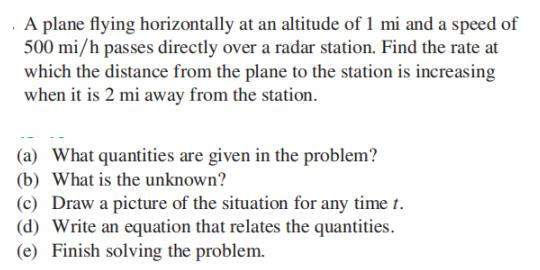### ¿Todavía tienes preguntas de matemáticas?

Pregunte a nuestros tutores expertos
Algebra
PreguntaA plane flying horizontally at an altitude of $$1 mi$$ and a speed of $$500 mi / h$$ passes directly over a radar station. Find the rate at which the distance from the plane to the station is increasing when it is $$2 mi$$ away from the station.

(a) What quantities are given in the problem? (b) What is the unknown? (c) Draw a picture of the situation for any time $$t$$ . (d) Write an equation that relates the quantities. (e) Finish solving the problem.

(a) The plane's altitude is $$1 mi$$ and its speed is $$500 mi / h$$ .
(b) The rate at which the distance from the plane to the station is increasing when the plane is $$2$$ mi from the station .
(d) $$y ^ { 2 } = x ^ { 2 } + 1$$
(e) $$250 \sqrt { 3 } mi / h$$